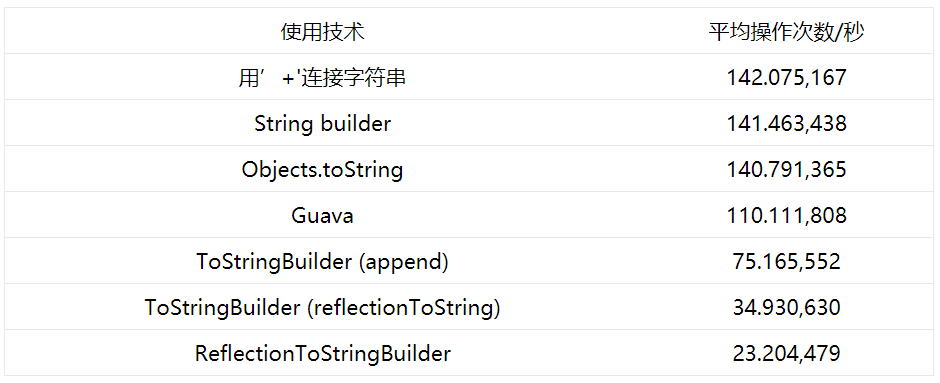# 誰在關心toString的性能？

（點擊

，可快速關注）

，所以這裡不再細談JMH如何工作的細節。

http://antoniogoncalves.org/2015/01/15/micro-benchmarking-with-jmh-measure-dont-guess/

public String toString() {

return "MyObject{" +

"att1="" + att1 + """ +

", att2="" + att2 + """ +

", att3="" + att3 + """ +

"} " + super.toString();

}

// Average performance with JMH (ops/s)

// (min, avg, max) = (140772,314, 142075,167, 143844,717)

// 使用JMH測出來的平均性能

// (最小, 平均, 最大) = (140772,314, 142075,167, 143844,717)

Java SE 7帶來了Objects類和它的一些靜態方法。Objects.toString的優點是它可以處理null值，甚至可以給null設置默認值。其性能與上一個相比略低，但是null值可以被處理：

public String toString() {

return "MyObject{" +

"att1="" + Objects.toString(att1) + """ +

", att2="" + Objects.toString(att2) + """ +

", att3="" + Objects.toString(att3) + """ +

"} " + super.toString();

}

// Average performance with JMH (ops/s)

// (min, avg, max) = (138790,233, 140791,365, 142031,847)

// 使用JMH測出來的平均性能

// (最小, 平均, 最大) = (138790,233, 140791,365, 142031,847)

StringBuilder

public String toString() {

final StringBuilder sb = new StringBuilder("MyObject{");

sb.append("att1="").append(att1).append(""");

sb.append(", att2="").append(att2).append(""");

sb.append(", att3="").append(att3).append(""");

sb.append(super.toString());

return sb.toString();

}

// Average performance with JMH (ops/s)

// (min, avg, max) = (96073,645, 141463,438, 146205,910)

// 使用JMH測出來的平均性能

// (最小, 平均, 最大) = (96073,645, 141463,438, 146205,910)

Guava

Guava有一些helper類：其中一個可以幫助你生成toString。這比純JDK API性能要差一點，但是它可以提供給你一些額外的服務（我這裡指的Guava）：

public String toString() {

return Objects.toStringHelper(this)

}

// Average performance with JMH (ops/s)

// (min, avg, max) = (97049,043, 110111,808, 114878,137)

// 使用JMH測出來的平均性能

// (最小, 平均, 最大) = (97049,043, 110111,808, 114878,137)

Commons Lang3

Commons Lang3有一些技術來生成toString：從builder到 introspector。如同你猜測到的，introspection更容易使用，代碼量更少，但是性能比較糟糕：

public String toString() {

return new ToStringBuilder(this)

.append("att1", att1)

.append("att2", att2)

.append("att3", att3)

.append("super", super.toString()).toString();

}

// Average performance with JMH (ops/s)

// (min, avg, max) = ( 73510,509,  75165,552,  76406,370)

// 使用JMH測出來的平均性能

// (最小, 平均, 最大) = ( 73510,509,  75165,552,  76406,370)

public String toString() {

}

// Average performance with JMH (ops/s)

// (min, avg, max) = (31803,224, 34930,630, 35581,488)

// 使用JMH測出來的平均性能

// (最小, 平均, 最大) =(31803,224, 34930,630, 35581,488)

public String toString() {

return ReflectionToStringBuilder.toString(this);

}

// Average performance with JMH (ops/s)

// (min, avg, max) = (14172,485, 23204,479, 30754,901)

// 使用JMH測出來的平均性能

// (最小, 平均, 最大) = (14172,485, 23204,479, 30754,901)TAG:ImportNew |